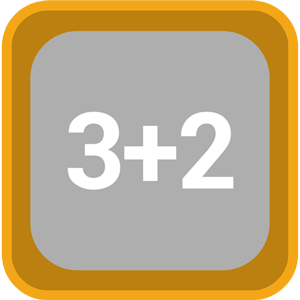# Voting Now

##### Creativity
×## Calculate the Squares

### by ArtoriusDesign

Free
Design
Usability
Creativity
Average
10 USERS VOTED
##### TAGS
###### Description
Calculate the Squares is the addicting math puzzle game that will test your math skills and luck in a math puzzle solving frenzy! It's very simple to play the game: you must clear the squares who have the same math result, for example: 3+2 and 1+4 or 4x2 and 5+3 FEATURES: - Infinite levels increasing in difficulty - Challenge your mind with all math operations: additions, substractions, multiplications, divisions and powers - Use powers to auto-solve all math operations on the board - Compete in multiplayer against facebook opponents Don't just push the squares, remember to calculate the squares! Math puzzle games will keep your brain alert and Calculate the Squares is a math puzzle game that will challenge you constantly because levels are infinite and are determined by difficulty settings, so if you retry the level, the squares will be different. This way, everytime, you will have to solve different math puzzles

View All